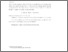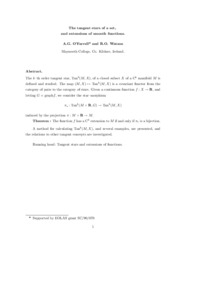# The tangent stars of a set, and extensions of smooth functions

Watson, R.O. and O'Farrell, Anthony G. (1992) The tangent stars of a set, and extensions of smooth functions. Journal fur die reine und angewandte Mathematik, 430. pp. 109-138. ISSN 0075-4102Previewmore...Add this article to your Mendeley library

## Abstract

The k–th order tangent star, Tank (M, X), of a closed subset X of a Ck manifold M is defined and studied. The map (M, X) 7→ Tank (M, X) is a covariant functor from the category of pairs to the category of stars. Given a continuous function f : X → R, and letting G = graphf, we consider the star–morphism π∗ : Tank (M × R, G) → Tank (M, X) induced by the projection π : M × R → M. Theorem : The function f has a Ck extension to M if and only if π∗ is a bijection. A method for calculating Tank (M, X), and several examples, are presented, and the relations to other tangent concepts are investigated.

Item Type: Article Cite as: Watson, R.O. and O'Farrell, A.G.. "The tangent stars of a set, and extensions of smooth functions.: " , vol. 1992, no. 430, 1992, pp. 109-138. https://doi.org/10.1515/crll.1992.430.109 tangent; stars; set; extensions; smooth functions; Faculty of Science and Engineering > Mathematics and Statistics 14704 https://doi.org/10.1515/crll.1992.430.109 Prof. Anthony O'Farrell 18 Aug 2021 14:21 Journal fur die reine und angewandte Mathematik de Gruyter YesItem control page